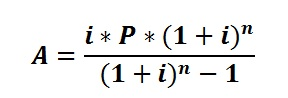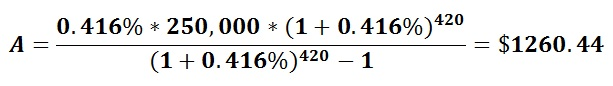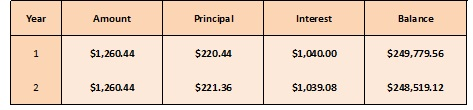# How to Calculate Amortization Schedule

Amortization schedule consists of a table or chart that shows each payment breakdown as the interest payments and the amount that goes forward as the principal balance. It also includes additional details regarding the amount of interest and principal already paid and the balance amount to be settled. These are commonly used in mortgages. But how to calculate amortization schedule? Here, a step by step approach to calculate amortization schedule is presented.

## Step by Step approach to calculate amortization schedule

Following example indicates the step by step approach to calculate amortization schedule. Let’s consider a person who is buying a new house for \$280,000 with a down payment of \$30,000. One of the banks agreed to provide \$250,000 mortgage at a fixed interest of 5% for 35 years. Then it is required to find out the monthly instalment together with the interest. It can be calculated in a step by step approach as shown below:

Step 1: Determine the total number of payments

With regard to the above example, it is required to do a monthly payment for 35 years. So the person needs to do (35*12) 420 payments throughout the mortgage.

Step 2: Determine the monthly payment

The bank is charging 5% annual interest rate and, therefore, the monthly rate would be 0.416% (5%/12). Following formula can be used in calculating the monthly payment.A = monthly payment
P = loan’s initial amount
i = monthly interest rate
n = total number of payments### Step 3: Determine the total interest

Then the total cost of the loan can be calculated since 420 payments of \$1260.44 to be made.

The total amount need to be paid = \$1260.44*420 = \$529,386.45

The original loan amount =\$ 529,386.45 – \$ 250,000.00 = \$279,386.45

It means that the person needs to pay almost double the loan amount at the end of thirty five years.

### Step 4: Determine the breakdown of each monthly payment

In order to determine the breakdown the monthly payment,

Monthly Interest Rate = \$250,000* 0.416% = \$1040.00

Mortgage Payment ={\$1260.44 – (250,000*0.416%)}= \$220.44

Balance amount to be paid after the first payment = (\$250,000 – \$220.44) = \$249,779.56

The second payment breakdown can be illustrated as follows:

(\$249,779.56*0.416%)= \$1039.08

Here, it is important to note that the interest payment for the mortgage will be decreased in a small percentage. That amount is (\$1040.00 – \$1039.08) = \$0.92

With this information, monthly amortization schedule can be prepared as below:This process of calculating interest on the remaining balance will continue until the mortgage is fully paid off. So the monthly interest payment reduces and the amount going to paying off the loan will increase. After 420 payments, the mortgage is fully settled.